# Access M/J Grade 6 Mathematics (#7812015)

This document was generated on CPALMS - www.cpalms.org
You are not viewing the current course, please click the current year’s tab.

#### Course Standards

Name Description
MA.6.AR.1.1: Given a mathematical or real-world context, translate written descriptions into algebraic expressions and translate algebraic expressions into written descriptions.
 Examples:The algebraic expression 7.2x-20 can be used to describe the daily profit of a company who makes \$7.20 per product sold with daily expenses of \$20.
##### Related Access Points
 Name Description MA.6.AR.1.AP.1: Write or select an algebraic expression that represents a real-world situation.

MA.6.AR.1.2:

Translate a real-world written description into an algebraic inequality in the form of a, a, ≥ a or ≤ a. Represent the inequality on a number line.

 Clarifications:Clarification 1: Variables may be on the left or right side of the inequality symbol.

 Examples:Mrs. Anna told her class that they will get a pizza if the class has an average of at least 83 out of 100 correct questions on the semester exam. The inequality g ≥ 83 can be used to represent the situation where students receive a pizza and the inequality g < 83 can be used to represent the situation where students do not receive a pizza.
##### Related Access Points
 Name Description MA.6.AR.1.AP.2: Write or select an inequality that represents a real-world situation.

MA.6.AR.1.3: Evaluate algebraic expressions using substitution and order of operations.
 Clarifications:Clarification 1: Within this benchmark, the expectation is to perform all operations with integers. Clarification 2: Refer to Properties of Operations, Equality and Inequality (Appendix D).

 Examples:Evaluate the expression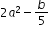, where a=-1 and b=15.
##### Related Access Points
 Name Description MA.6.AR.1.AP.3: Solve an expression using substitution with no more than two operations.

MA.6.AR.1.4: Apply the properties of operations to generate equivalent algebraic expressions with integer coefficients.
 Clarifications:Clarification 1: Properties include associative, commutative and distributive. Clarification 2: Refer to Properties of Operations, Equality and Inequality (Appendix D).

 Examples:Example: The expression 5(3x+1) can be rewritten equivalently as 15x+5. Example: If the expression 2x+3x represents the profit the cheerleading team can make when selling the same number of cupcakes, sold for \$2 each, and brownies, sold for \$3 each. The expression 5x can express the total profit.
##### Related Access Points
 Name Description MA.6.AR.1.AP.4: Use tools or models to combine like terms in an expression with no more than four operations.

MA.6.AR.2.1: Given an equation or inequality and a specified set of integer values, determine which values make the equation or inequality true or false.
 Clarifications:Clarification 1: Problems include the variable in multiple terms or on either side of the equal sign or inequality symbol.

 Examples:Determine which of the following values make the inequality x+1<2 true: -4,-2,0,1.
##### Related Access Points
 Name Description MA.6.AR.2.AP.1: Choose which values, from a set of five or fewer integers, make an equation or inequality true.

MA.6.AR.2.2: Write and solve one-step equations in one variable within a mathematical or real-world context using addition and subtraction, where all terms and solutions are integers.
 Clarifications:Clarification 1: Instruction includes using manipulatives, drawings, number lines and inverse operations. Clarification 2: Instruction includes equations in the forms x+p=q and p+x=q, where x,p and q are any integer. Clarification 3: Problems include equations where the variable may be on either side of the equal sign.

 Examples:The equations -35+x=17, 17=-35+x and 17-x=-35 can represent the question “How many units to the right is 17 from -35 on the number line?”
##### Related Access Points
 Name Description MA.6.AR.2.AP.2: Solve real-world, one-step linear equations using addition and subtraction involving integers.

MA.6.AR.2.3: Write and solve one-step equations in one variable within a mathematical or real-world context using multiplication and division, where all terms and solutions are integers.
 Clarifications:Clarification 1: Instruction includes using manipulatives, drawings, number lines and inverse operations. Clarification 2: Instruction includes equations in the forms x/p = q, where p ≠ 0, and px = q. Clarification 3: Problems include equations where the variable may be on either side of the equal sign.
##### Related Access Points
 Name Description MA.6.AR.2.AP.3: Solve real-world, one-step linear equations using multiplication and division involving integers.

MA.6.AR.2.4: Determine the unknown decimal or fraction in an equation involving any of the four operations, relating three numbers, with the unknown in any position.
 Clarifications:Clarification 1: Instruction focuses on using algebraic reasoning, drawings, and mental math to determine unknowns. Clarification 2: Problems include the unknown and different operations on either side of the equal sign. All terms and solutions are limited to positive rational numbers.

 Examples:Given the equation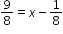, x can be determined to be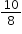becauseis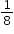more than.
##### Related Access Points
 Name Description MA.6.AR.2.AP.4: Solve a one-step equation using fractions with like denominators or decimals with place value ranging from the thousand to the thousandths.

MA.6.AR.3.1: Given a real-world context, write and interpret ratios to show the relative sizes of two quantities using appropriate notation:, a to b, or a:b where b ≠ 0.
 Clarifications:Clarification 1: Instruction focuses on the understanding that a ratio can be described as a comparison of two quantities in either the same or different units. Clarification 2: Instruction includes using manipulatives, drawings, models and words to interpret part-to-part ratios and part-to-whole ratios. Clarification 3: The values of a and b are limited to whole numbers.
##### Related Access Points
 Name Description MA.6.AR.3.AP.1: Given a real-world context, write and interpret ratios to show the relative sizes of two quantities using notation: a/b, a to b, or a:b where b ≠ 0 with guidance and support.

MA.6.AR.3.2: Given a real-world context, determine a rate for a ratio of quantities with different units. Calculate and interpret the corresponding unit rate.
 Clarifications:Clarification 1: Instruction includes using manipulatives, drawings, models and words and making connections between ratios, rates and unit rates. Clarification 2: Problems will not include conversions between customary and metric systems.

 Examples:Tamika can read 500 words in 3 minutes. Her reading rate can be described as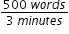which is equivalent to the unit rate of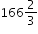words per minute.
##### Related Access Points
 Name Description MA.6.AR.3.AP.2: Given a rate, calculate the unit rate for a ratio with different units.

MA.6.AR.3.3: Extend previous understanding of fractions and numerical patterns to generate or complete a two- or three-column table to display equivalent part-to-part ratios and part-to-part-to-whole ratios.
 Clarifications:Clarification 1: Instruction includes using two-column tables (e.g., a relationship between two variables) and three-column tables (e.g., part-to-part-to-whole relationship) to generate conversion charts and mixture charts.

Examples:
The table below expresses the relationship between the number of ounces of yellow and blue paints used to create a new color. Determine the ratios and complete the table.

 Yellow (part) 1.5 3 9 Blue (part) 2 4 New Color (whole) 12 21

##### Related Access Points
 Name Description MA.6.AR.3.AP.3: Given a visual representation, write or select a ratio that describes the ratio relationship between part-to-part and part-to-whole ratios.

MA.6.AR.3.4: Apply ratio relationships to solve mathematical and real-world problems involving percentages using the relationship between two quantities.
 Clarifications:Clarification 1: Instruction includes using manipulatives, drawings, number lines and inverse operations. Clarification 2: Instruction includes equations in the forms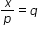, where p≠0, and px=q. Clarification 3: Problems include equations where the variable may be on either side of the equal sign.

 Examples:Gerald is trying to gain muscle and needs to consume more protein every day. If he has a protein shake that contain 32 grams and the entire shake is 340 grams, what percentage of the entire shake is protein? What is the ratio between grams of protein and grams of non-protein?
##### Related Access Points
 Name Description MA.6.AR.3.AP.4: Calculate a percentage of quantity as rate per 100 using models (e.g., percent bars or 10 × 10 grids).

MA.6.AR.3.5: Solve mathematical and real-world problems involving ratios, rates and unit rates, including comparisons, mixtures, ratios of lengths and conversions within the same measurement system.
 Clarifications:Clarification 1: Instruction includes the use of tables, tape diagrams and number lines.
##### Related Access Points
 Name Description MA.6.AR.3.AP.5a: Use tools, models or manipulatives to solve problems involving ratio relationships including mixtures and ratios of length. MA.6.AR.3.AP.5b: Use tools, models or manipulatives to solve ratio, rate or unit rate problems involving conversions within the same measurement system.

MA.6.DP.1.1: Recognize and formulate a statistical question that would generate numerical data.
 Examples:The question “How many minutes did you spend on mathematics homework last night?” can be used to generate numerical data in one variable.
##### Related Access Points
 Name Description MA.6.DP.1.AP.1: Identify statistical questions from a list that would generate numerical data.

MA.6.DP.1.2: Given a numerical data set within a real-world context, find and interpret mean, median, mode and range.
 Clarifications:Clarification 1: Numerical data is limited to positive rational numbers.

 Examples:The data set {15,0,32,24,0,17,42,0,29,120,0,20}, collected based on minutes spent on homework, has a mode of 0.
##### Related Access Points
 Name Description MA.6.DP.1.AP.2a: Use tools to identify and calculate the mean, median, mode and range represented in a set of data with no more than five elements. MA.6.DP.1.AP.2b: Identify and explain what the mean and mode represent in a set of data with no more than five elements.

MA.6.DP.1.3: Given a box plot within a real-world context, determine the minimum, the lower quartile, the median, the upper quartile and the maximum. Use this summary of the data to describe the spread and distribution of the data.
 Clarifications:Clarification 1: Instruction includes describing range, interquartile range, halves and quarters of the data.

 Examples:The middle 50% of the population can be determined by finding the interval between the upper quartile and the lower quartile.
##### Related Access Points
 Name Description MA.6.DP.1.AP.3: Given a box plot, identify the value of the minimum, the lower quartile, the median, the upper quartile and the maximum.

MA.6.DP.1.4: Given a histogram or line plot within a real-world context, qualitatively describe and interpret the spread and distribution of the data, including any symmetry, skewness, gaps, clusters, outliers and the range.
 Clarifications:Clarification 1: Refer to K-12 Mathematics Glossary (Appendix C).
##### Related Access Points
 Name Description MA.6.DP.1.AP.4: Given a histogram or a line plot, describe the physical features of the graph.

MA.6.DP.1.5: Create box plots and histograms to represent sets of numerical data within real-world contexts.
 Clarifications:Clarification 1: Instruction includes collecting data and discussing ways to collect truthful data to construct graphical representations. Clarification 2: Within this benchmark, it is the expectation to use appropriate titles, labels, scales and units when constructing graphical representations. Clarification 3: Numerical data is limited to positive rational numbers.

 Examples:The numerical data set {15,0,32,24,0,17,42,0,29,120,0,20}, collected based on minutes spent on homework, can be represented graphically using a box plot.
##### Related Access Points
 Name Description MA.6.DP.1.AP.5: Create histograms to represent sets of numerical data with 10 or fewer elements.

MA.6.DP.1.6: Given a real-world scenario, determine and describe how changes in data values impact measures of center and variation.
 Clarifications:Clarification 1: Instruction includes choosing the measure of center or measure of variation depending on the scenario. Clarification 2: The measures of center are limited to mean and median. The measures of variation are limited to range and interquartile range. Clarification 3: Numerical data is limited to positive rational numbers.
##### Related Access Points
 Name Description MA.6.DP.1.AP.6: Calculate and identify changes (increase or decrease) in the median, mode or range when a data value is added or subtracted from a data set.

MA.6.GR.1.1: Extend previous understanding of the coordinate plane to plot rational number ordered pairs in all four quadrants and on both axes. Identify the x- or y-axis as the line of reflection when two ordered pairs have an opposite x- or y-coordinate.
##### Related Access Points
 Name Description MA.6.GR.1.AP.1: Plot integer ordered pairs in all four quadrants and on both axes.

MA.6.GR.1.2: Find distances between ordered pairs, limited to the same x-coordinate or the same y-coordinate, represented on the coordinate plane.
##### Related Access Points
 Name Description MA.6.GR.1.AP.2: Count the distance between two ordered pairs with the same x-coordinate or the same y-coordinate.

MA.6.GR.1.3: Solve mathematical and real-world problems by plotting points on a coordinate plane, including finding the perimeter or area of a rectangle.
 Clarifications:Clarification 1: Instruction includes finding distances between points, computing dimensions of a rectangle or determining a fourth vertex of a rectangle. Clarification 2: Problems involving rectangles are limited to cases where the sides are parallel to the axes.
##### Related Access Points
 Name Description MA.6.GR.1.AP.3: Given a rectangle plotted on the coordinate plane, find the perimeter or area of the rectangle.

MA.6.GR.2.1: Derive a formula for the area of a right triangle using a rectangle. Apply a formula to find the area of a triangle.
 Clarifications:Clarification 1: Instruction focuses on the relationship between the area of a rectangle and the area of a right triangle. Clarification 2: Within this benchmark, the expectation is to know from memory a formula for the area of a triangle.
##### Related Access Points
 Name Description MA.6.GR.2.AP.1: Given the formula, find the area of a triangle.

MA.6.GR.2.2: Solve mathematical and real-world problems involving the area of quadrilaterals and composite figures by decomposing them into triangles or rectangles.
 Clarifications:Clarification 1: Problem types include finding area of composite shapes and determining missing dimensions. Clarification 2: Within this benchmark, the expectation is to know from memory a formula for the area of a rectangle and triangle. Clarification 3: Dimensions are limited to positive rational numbers.
##### Related Access Points
 Name Description MA.6.GR.2.AP.2: Decompose quadrilaterals and composite figures into simple shapes (rectangles or triangles) to measure area.

MA.6.GR.2.3: Solve mathematical and real-world problems involving the volume of right rectangular prisms with positive rational number edge lengths using a visual model and a formula.
 Clarifications:Clarification 1: Problem types include finding the volume or a missing dimension of a rectangular prism.
##### Related Access Points
 Name Description MA.6.GR.2.AP.3: Given a real-world problem, find the volume of a rectangular prism using a visual model and the formula.

MA.6.GR.2.4: Given a mathematical or real-world context, find the surface area of right rectangular prisms and right rectangular pyramids using the figure’s net.
 Clarifications:Clarification 1: Instruction focuses on representing a right rectangular prism and right rectangular pyramid with its net and on the connection between the surface area of a figure and its net. Clarification 2: Within this benchmark, the expectation is to find the surface area when given a net or when given a three-dimensional figure. Clarification 3: Problems involving right rectangular pyramids are limited to cases where the heights of triangles are given. Clarification 4: Dimensions are limited to positive rational numbers.
##### Related Access Points
 Name Description MA.6.GR.2.AP.4: Find the surface area of right rectangular prisms by adding the areas of the shapes forming the two-dimensional net.

MA.6.NSO.1.1: Extend previous understanding of numbers to define rational numbers. Plot, order and compare rational numbers.
 Clarifications:Clarification 1: Within this benchmark, the expectation is to plot, order and compare positive and negative rational numbers when given in the same form and to plot, order and compare positive rational numbers when given in different forms (fraction, decimal, percentage). Clarification 2: Within this benchmark, the expectation is to use symbols (<, > or =).
##### Related Access Points
 Name Description MA.6.NSO.1.AP.1: Plot, order and compare rational numbers (positive and negative integers within 10 from 0, fractions with common denominators, decimals up to the hundredths and percentages) in the same form.

MA.6.NSO.1.2: Given a mathematical or real-world context, represent quantities that have opposite direction using rational numbers. Compare them on a number line and explain the meaning of zero within its context.
 Clarifications:Clarification 1: Instruction includes vertical and horizontal number lines, context referring to distances, temperatures and finances and using informal verbal comparisons, such as, lower, warmer or more in debt.Clarification 2: Within this benchmark, the expectation is to compare positive and negative rational numbers when given in the same form.

 Examples:Jasmine is on a cruise and is going on a scuba diving excursion. Her elevations of 10 feet above sea level and 8 feet below sea level can be compared on a number line, where 0 represents sea level.
##### Related Access Points
 Name Description MA.6.NSO.1.AP.2: Represent positive and negative numbers in the same form on a number line given a real-world situation and explain the meaning of zero within its context.

MA.6.NSO.1.3: Given a mathematical or real-world context, interpret the absolute value of a number as the distance from zero on a number line. Find the absolute value of rational numbers.
 Clarifications:Clarification 1: Instruction includes the connection of absolute value to mirror images about zero and to opposites. Clarification 2: Instruction includes vertical and horizontal number lines and context referring to distances, temperature and finances.
##### Related Access Points
 Name Description MA.6.NSO.1.AP.3: Find absolute value of a rational number ranging from –30 to 30 using a number line.

MA.6.NSO.1.4: Solve mathematical and real-world problems involving absolute value, including the comparison of absolute value.
 Clarifications:Clarification 1: Absolute value situations include distances, temperatures and finances. Clarification 2: Problems involving calculations with absolute value are limited to two or fewer operations. Clarification 3: Within this benchmark, the expectation is to use integers only.

 Examples:Michael has a lemonade stand which costs \$10 to start up. If he makes \$5 the first day, he can determine whether he made a profit so far by comparing |-10| and |5|.
##### Related Access Points
 Name Description MA.6.NSO.1.AP.4: Use manipulatives, models or tools to compare absolute value in mathematical and real-world problems.

MA.6.NSO.2.1: Multiply and divide positive multi-digit numbers with decimals to the thousandths, including using a standard algorithm with procedural fluency.
 Clarifications:Clarification 1: Multi-digit decimals are limited to no more than 5 total digits.
##### Related Access Points
 Name Description MA.6.NSO.2.AP.1: Solve one-step multiplication and division problems involving positive decimals whose place value ranges from the tens to the hundredths places.

MA.6.NSO.2.2: Extend previous understanding of multiplication and division to compute products and quotients of positive fractions by positive fractions, including mixed numbers, with procedural fluency.
 Clarifications:Clarification 1: Instruction focuses on making connections between visual models, the relationship between multiplication and division, reciprocals and algorithms.
##### Related Access Points
 Name Description MA.6.NSO.2.AP.2: Use tools to calculate the product and quotient of positive fractions by positive fractions, including mixed numbers, using the standard algorithms.

MA.6.NSO.2.3: Solve multi-step real-world problems involving any of the four operations with positive multi-digit decimals or positive fractions, including mixed numbers.
 Clarifications:Clarification 1: Within this benchmark, it is not the expectation to include both decimals and fractions within a single problem.
##### Related Access Points
 Name Description MA.6.NSO.2.AP.3a: Solve one-step real-world problems involving any of the four operations with positive decimals ranging from the hundreds to hundredth place value. MA.6.NSO.2.AP.3b: Solve one-step real-world problems involving any of the four operations with positive fractions and mixed numbers with like denominators.

MA.6.NSO.3.1: Given a mathematical or real-world context, find the greatest common factor and least common multiple of two whole numbers.
 Clarifications:Clarification 1: Within this benchmark, expectations include finding greatest common factor within 1,000 and least common multiple with factors to 25.Clarification 2: Instruction includes finding the greatest common factor of the numerator and denominator of a fraction to simplify a fraction.

 Examples:Example: Middleton Middle School’s band has an upcoming winter concert which will have several performances. The bandleader would like to divide the students into concert groups with the same number of flute players, the same number of clarinet players and the same number of violin players in each group. There are a total of 15 students who play the flute, 27 students who play the clarinet and 12 students who play the violin. How many separate groups can be formed? Example: Adam works out every 8 days and Susan works out every 12 days. If both Adam and Susan work out today, how many days until they work out on the same day again?
##### Related Access Points
 Name Description MA.6.NSO.3.AP.1: Use tools to find the greatest common factor and least common multiple of two whole numbers 50 or less.

MA.6.NSO.3.2: Rewrite the sum of two composite whole numbers having a common factor, as a common factor multiplied by the sum of two whole numbers.
 Clarifications:Clarification 1: Instruction includes using the distributive property to generate equivalent expressions.
##### Related Access Points
 Name Description MA.6.NSO.3.AP.2: Use the distributive property to express a number as the sum of two whole numbers multiplied by a common factor.

MA.6.NSO.3.3: Evaluate positive rational numbers and integers with natural number exponents.
 Clarifications:Clarification 1: Within this benchmark, expectations include using natural number exponents up to 5.
##### Related Access Points
 Name Description MA.6.NSO.3.AP.3a: Identify what an exponent represents (e.g., 8³= 8 × 8 × 8). MA.6.NSO.3.AP.3b: Solve numerical expressions involving whole-number bases and exponents (e.g.,5+×6=101).

MA.6.NSO.3.4: Express composite whole numbers as a product of prime factors with natural number exponents.
##### Related Access Points
 Name Description MA.6.NSO.3.AP.4: 4 Use a tool to show the prime factors of a composite whole number (e.g., 20 = 2 × 2 × 5).

MA.6.NSO.3.5: Rewrite positive rational numbers in different but equivalent forms including fractions, terminating decimals and percentages.
 Clarifications:Clarification 1: Rational numbers include decimal equivalence up to the thousandths place.

 Examples:The number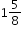can be written equivalently as 1.625 or 162.5%
##### Related Access Points
 Name Description MA.6.NSO.3.AP.5: Rewrite a positive rational number 3 or less, as a fraction, decimal or a percent.

MA.6.NSO.4.1: Apply and extend previous understandings of operations with whole numbers to add and subtract integers with procedural fluency.
 Clarifications:Clarification 1: Instruction begins with the use of manipulatives, models and number lines working towards becoming procedurally fluent by the end of grade 6. Clarification 2: Instruction focuses on the inverse relationship between the operations of addition and subtraction. If p and q are integers, then p-q=p+(-q) and p+q=p-(-q).
##### Related Access Points
 Name Description MA.6.NSO.4.AP.1: Use tools to add and subtract integers between 50 and −50.

MA.6.NSO.4.2: Apply and extend previous understandings of operations with whole numbers to multiply and divide integers with procedural fluency.
 Clarifications:Clarification 1: Instruction includes the use of models and number lines and the inverse relationship between multiplication and division, working towards becoming procedurally fluent by the end of grade 6. Clarification 2: Instruction focuses on the understanding that integers can be divided, provided that the divisor is not zero, and every quotient of integers (with non-zero divisor) is a rational number. If p and q are integers where q≠0, then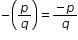,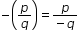and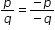.
##### Related Access Points
 Name Description MA.6.NSO.4.AP.2: Use tools to multiply and divide integers between 20 and −20.

MA.K12.MTR.1.1: Actively participate in effortful learning both individually and collectively.

Mathematicians who participate in effortful learning both individually and with others:

• Analyze the problem in a way that makes sense given the task.
• Build perseverance by modifying methods as needed while solving a challenging task.
• Stay engaged and maintain a positive mindset when working to solve tasks.
• Help and support each other when attempting a new method or approach.

 Clarifications:Teachers who encourage students to participate actively in effortful learning both individually and with others: Cultivate a community of growth mindset learners.  Foster perseverance in students by choosing tasks that are challenging. Develop students’ ability to analyze and problem solve. Recognize students’ effort when solving challenging problems.
MA.K12.MTR.2.1: Demonstrate understanding by representing problems in multiple ways.

Mathematicians who demonstrate understanding by representing problems in multiple ways:

• Build understanding through modeling and using manipulatives.
• Represent solutions to problems in multiple ways using objects, drawings, tables, graphs and equations.
• Progress from modeling problems with objects and drawings to using algorithms and equations.
• Express connections between concepts and representations.
• Choose a representation based on the given context or purpose.
 Clarifications:Teachers who encourage students to demonstrate understanding by representing problems in multiple ways: Help students make connections between concepts and representations.Provide opportunities for students to use manipulatives when investigating concepts.Guide students from concrete to pictorial to abstract representations as understanding progresses.Show students that various representations can have different purposes and can be useful in different situations.
MA.K12.MTR.3.1: Complete tasks with mathematical fluency.

Mathematicians who complete tasks with mathematical fluency:

• Select efficient and appropriate methods for solving problems within the given context.
• Maintain flexibility and accuracy while performing procedures and mental calculations.
• Complete tasks accurately and with confidence.
• Adapt procedures to apply them to a new context.
• Use feedback to improve efficiency when performing calculations.
 Clarifications:Teachers who encourage students to complete tasks with mathematical fluency:Provide students with the flexibility to solve problems by selecting a procedure that allows them to solve efficiently and accurately.Offer multiple opportunities for students to practice efficient and generalizable methods.Provide opportunities for students to reflect on the method they used and determine if a more efficient method could have been used.
MA.K12.MTR.4.1: Engage in discussions that reflect on the mathematical thinking of self and others.

Mathematicians who engage in discussions that reflect on the mathematical thinking of self and others:

• Communicate mathematical ideas, vocabulary and methods effectively.
• Analyze the mathematical thinking of others.
• Compare the efficiency of a method to those expressed by others.
• Recognize errors and suggest how to correctly solve the task.
• Justify results by explaining methods and processes.
• Construct possible arguments based on evidence.
 Clarifications:Teachers who encourage students to engage in discussions that reflect on the mathematical thinking of self and others:Establish a culture in which students ask questions of the teacher and their peers, and error is an opportunity for learning.Create opportunities for students to discuss their thinking with peers.Select, sequence and present student work to advance and deepen understanding of correct and increasingly efficient methods.Develop students’ ability to justify methods and compare their responses to the responses of their peers.
MA.K12.MTR.5.1: Use patterns and structure to help understand and connect mathematical concepts.

Mathematicians who use patterns and structure to help understand and connect mathematical concepts:

• Focus on relevant details within a problem.
• Create plans and procedures to logically order events, steps or ideas to solve problems.
• Decompose a complex problem into manageable parts.
• Relate previously learned concepts to new concepts.
• Look for similarities among problems.
• Connect solutions of problems to more complicated large-scale situations.
 Clarifications:Teachers who encourage students to use patterns and structure to help understand and connect mathematical concepts:Help students recognize the patterns in the world around them and connect these patterns to mathematical concepts.Support students to develop generalizations based on the similarities found among problems.Provide opportunities for students to create plans and procedures to solve problems.Develop students’ ability to construct relationships between their current understanding and more sophisticated ways of thinking.
MA.K12.MTR.6.1: Assess the reasonableness of solutions.

Mathematicians who assess the reasonableness of solutions:

• Estimate to discover possible solutions.
• Use benchmark quantities to determine if a solution makes sense.
• Check calculations when solving problems.
• Verify possible solutions by explaining the methods used.
• Evaluate results based on the given context.
 Clarifications:Teachers who encourage students to assess the reasonableness of solutions:Have students estimate or predict solutions prior to solving.Prompt students to continually ask, “Does this solution make sense? How do you know?”Reinforce that students check their work as they progress within and after a task.Strengthen students’ ability to verify solutions through justifications.
MA.K12.MTR.7.1: Apply mathematics to real-world contexts.

Mathematicians who apply mathematics to real-world contexts:

• Connect mathematical concepts to everyday experiences.
• Use models and methods to understand, represent and solve problems.
• Perform investigations to gather data or determine if a method is appropriate. • Redesign models and methods to improve accuracy or efficiency.
 Clarifications:Teachers who encourage students to apply mathematics to real-world contexts:Provide opportunities for students to create models, both concrete and abstract, and perform investigations.Challenge students to question the accuracy of their models and methods.Support students as they validate conclusions by comparing them to the given situation.Indicate how various concepts can be applied to other disciplines.
ELA.K12.EE.1.1: Cite evidence to explain and justify reasoning.
 Clarifications:K-1 Students include textual evidence in their oral communication with guidance and support from adults. The evidence can consist of details from the text without naming the text. During 1st grade, students learn how to incorporate the evidence in their writing.2-3 Students include relevant textual evidence in their written and oral communication. Students should name the text when they refer to it. In 3rd grade, students should use a combination of direct and indirect citations.4-5 Students continue with previous skills and reference comments made by speakers and peers. Students cite texts that they’ve directly quoted, paraphrased, or used for information. When writing, students will use the form of citation dictated by the instructor or the style guide referenced by the instructor. 6-8 Students continue with previous skills and use a style guide to create a proper citation.9-12 Students continue with previous skills and should be aware of existing style guides and the ways in which they differ.
 Clarifications:See Text Complexity for grade-level complexity bands and a text complexity rubric.
ELA.K12.EE.3.1: Make inferences to support comprehension.
 Clarifications:Students will make inferences before the words infer or inference are introduced. Kindergarten students will answer questions like “Why is the girl smiling?” or make predictions about what will happen based on the title page. Students will use the terms and apply them in 2nd grade and beyond.
ELA.K12.EE.4.1: Use appropriate collaborative techniques and active listening skills when engaging in discussions in a variety of situations.
 Clarifications:In kindergarten, students learn to listen to one another respectfully.In grades 1-2, students build upon these skills by justifying what they are thinking. For example: “I think ________ because _______.” The collaborative conversations are becoming academic conversations.In grades 3-12, students engage in academic conversations discussing claims and justifying their reasoning, refining and applying skills. Students build on ideas, propel the conversation, and support claims and counterclaims with evidence.
ELA.K12.EE.5.1: Use the accepted rules governing a specific format to create quality work.
 Clarifications:Students will incorporate skills learned into work products to produce quality work. For students to incorporate these skills appropriately, they must receive instruction. A 3rd grade student creating a poster board display must have instruction in how to effectively present information to do quality work.
ELA.K12.EE.6.1: Use appropriate voice and tone when speaking or writing.
 Clarifications:In kindergarten and 1st grade, students learn the difference between formal and informal language. For example, the way we talk to our friends differs from the way we speak to adults. In 2nd grade and beyond, students practice appropriate social and academic language to discuss texts.
ELD.K12.ELL.MA.1: English language learners communicate information, ideas and concepts necessary for academic success in the content area of Mathematics.
ELD.K12.ELL.SI.1: English language learners communicate for social and instructional purposes within the school setting.

## General Course Information and Notes

### VERSION DESCRIPTION

Access Courses:

Access courses are for students with the most significant cognitive disabilities. Access courses are designed to provide students access to grade-level general curriculum. Access points are alternate academic achievement standards included in access courses that target the salient content of Florida’s standards. Access points are intentionally designed to academically challenge students with the most significant cognitive disabilities.

### GENERAL NOTES

English Language Development ELD Standards Special Notes Section:

Teachers are required to provide listening, speaking, reading and writing instruction that allows English language learners (ELL) to communicate information, ideas and concepts for academic success in the content area of Language Arts. For the given level of English language proficiency and with visual, graphic, or interactive support, students will interact with grade level words, expressions, sentences and discourse to process or produce language necessary for academic success. The ELD standard should specify a relevant content area concept or topic of study chosen by curriculum developers and teachers which maximizes an ELL's need for communication and social skills. To access an ELL supporting document which delineates performance definitions and descriptors, please click on the following link: https://cpalmsmediaprod.blob.core.windows.net/uploads/docs/standards/eld/la.pdf.

### General Information

 Course Number: 7812015 Course Path: Section: Exceptional Student Education > Grade Group: Middle/Junior High > Subject: Academics - Subject Areas > Abbreviated Title: ACCESS M/J GR 6 MATH Course Attributes: Class Size Core Required Florida Standards Course Course Type: Core Academic Course Course Status: Course Approved